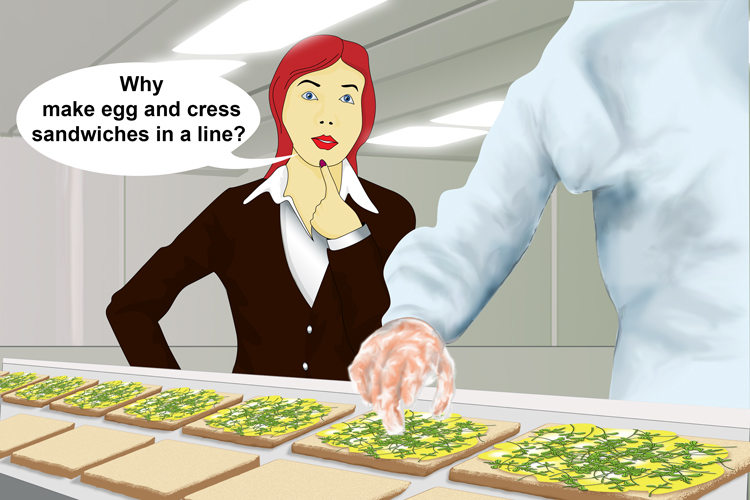# Remember the formula for a straight line

To remember that y=mx+cShe formed a straight line (formula for a straight line) of sandwiches.Why (y) have you made (m) egg (x) and (+) cress (c) sandwiches in a line?

Formula for a straight line:

 Why (y) have you made (m) rarr y\ \mx+c Egg (x) and (+) cress (c) = y=mx+c